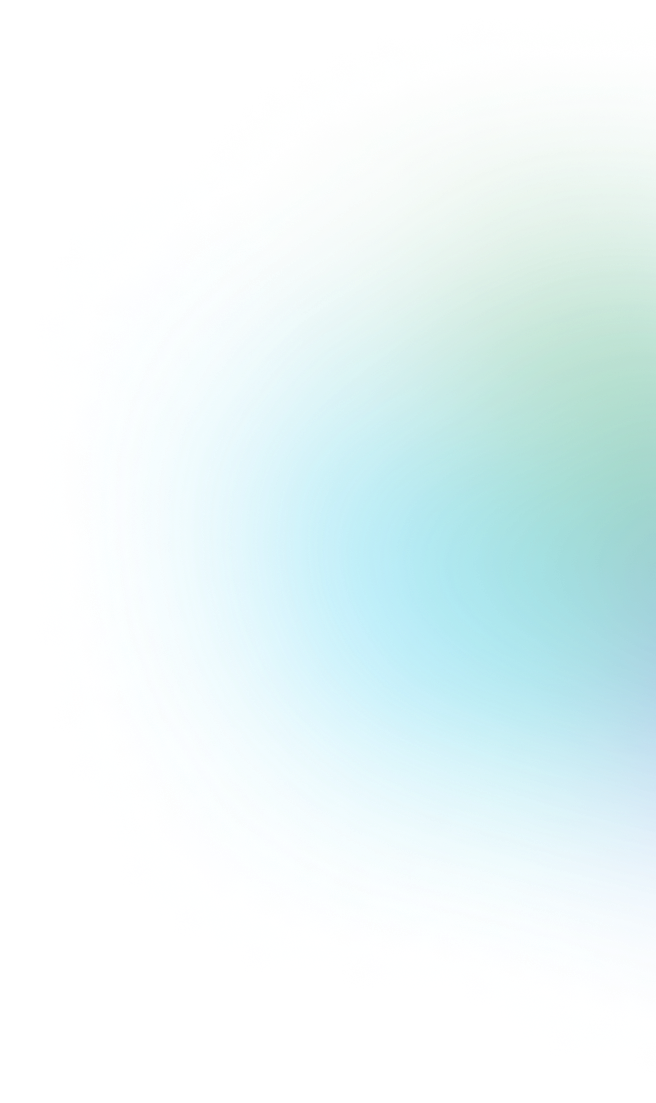Back
Blog Post
|
#engineering

# Binary trees, what are they?Usman Ibrahim
November 29, 2021I can still recall my introduction to the “Binary Tree’’ as a data structure; the peculiarity of its name sprung an immediate interest in me. Nonetheless, I began personal research and was quickly unmotivated due to the density of the material at the time. Thus, I searched for and discovered alternative ways to master content on this subject.

Before I continue a discussion on Binary Tree’s, I’d like to define the key terms necessary for its understanding.

### Branch

A part of a tree that grows out from the trunk or another branch.

### Node

Put simply, A node is the beginning or the ending of a branch in a tree. I.e a node connects different branches of a tree. Nodes connect branches of a tree meaning they exist at both the end and beginning of a branch; thus, with every node, there is at least one branch.

### Root Node

The root of a tree is the foundation of the tree. Understood as the origin or starting point, all other parts of the tree (nodes through branches) emerge from it. Fundamentally, the root node is unique and has no branching leading to it.

### Child Node

A child node is a node that descends from another node through a branch. Two nodes that descend from the same node are called sibling nodes.

### Parent Node

A parent node is a node that has other nodes on its branches. The root node of a tree, for example, is a parent of the immediate nodes that branch out of it!.

### Leaf Node

A leaf node is a terminal node having no further branches or nodes emerging from it. If the root node is the head of a tree, leaf nodes are the tail ends of the tree.

### Binary

When we hear the word binary, the number two comes to mind. That is, a binary entails anything that is related to or involves two parts. Simply, a binary entity can assume only either of any two options. Examples of binary options include: True or False, Yes or No, and 0 or 1.

Okay, enough talk about nodes, look at the beautiful tree picture below, can you identify all the terms defined above?. I now want you to make an educated guess on what you think a binary tree is, before reading the next paragraph.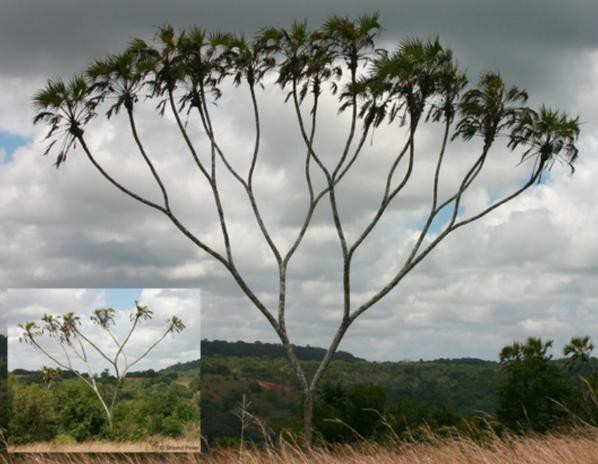Fig 1: Real Binary Tree

## Binary Tree

As you might have guessed, A binary tree is a tree with a maximum of two child nodes for each parent node. A binary tree is also a non-linear data structure where each node represents an element, that encloses some value and references at most two child nodes. We have a tree structure in Fig 2 that depicts our real binary tree when viewed upside down.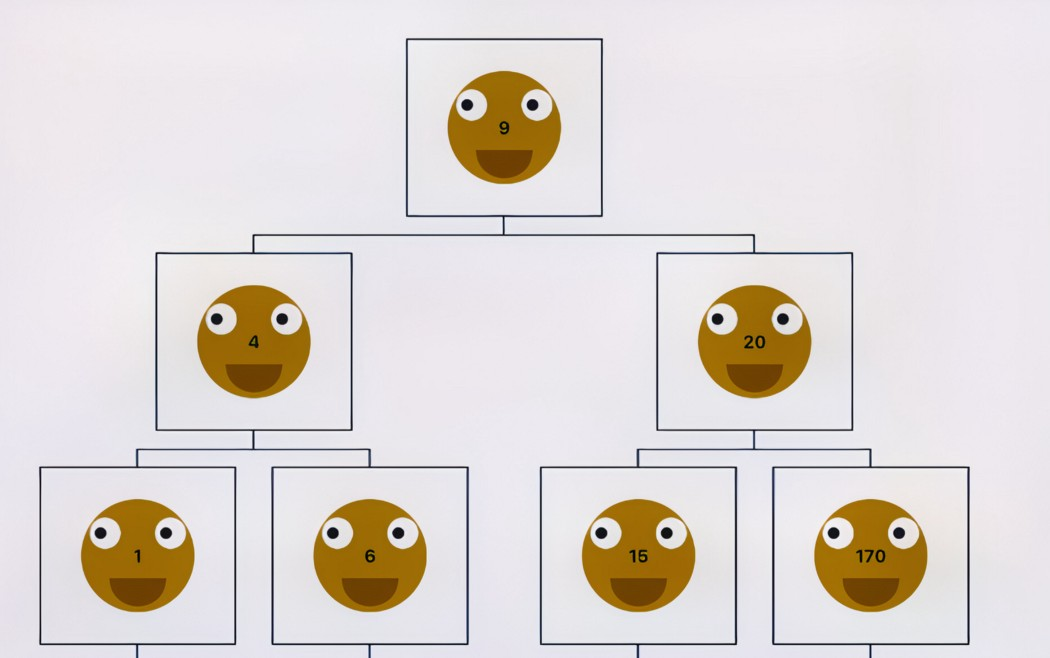### Types of a binary tree

Having established that any parent node can only have a maximum of two child nodes; there are various types of binary trees. Often distinguished by either the number of child nodes or the arrangement of the nodes in the tree.

#### Full Binary Tree

A full binary tree is a type of binary tree in which each node has an even number of nodes. And since a parent node can have at most two child nodes, the number of child nodes of a full binary tree can only be zero or two. A full binary tree is depicted in Fig. 3.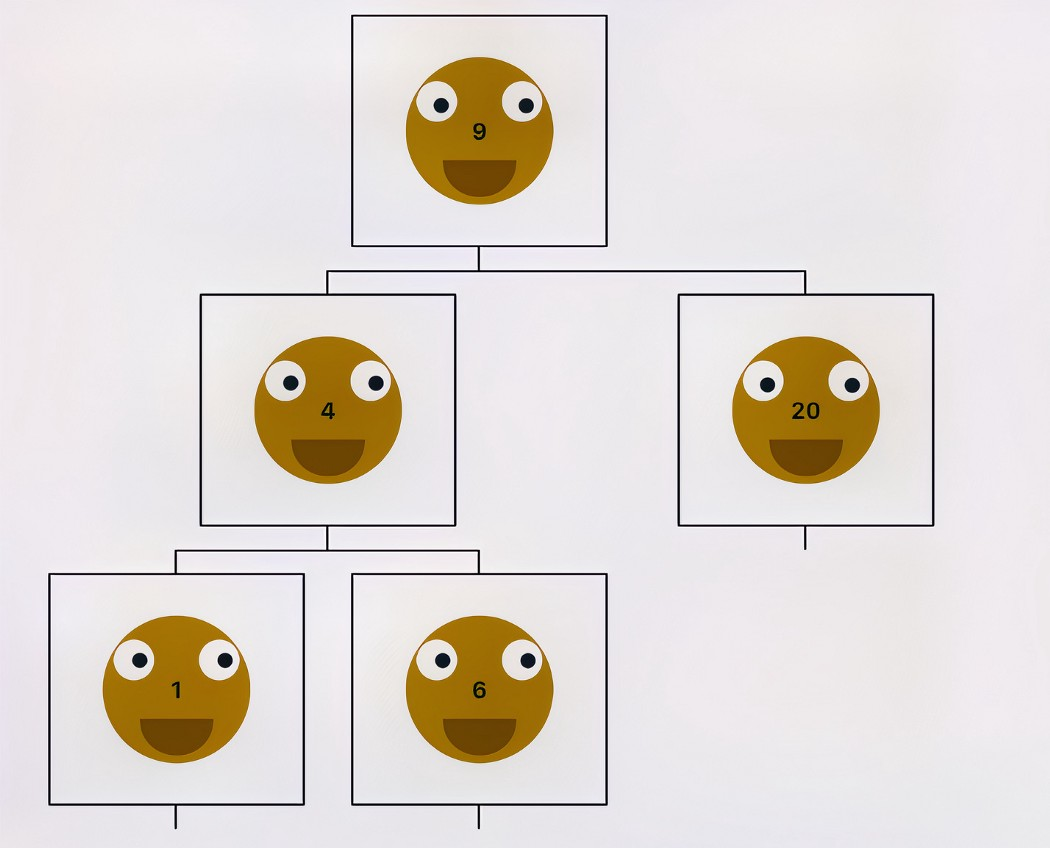#### Complete Binary Tree

A complete binary tree is a tree in which each level is filled with nodes while descending the tree except possibly the lowest level. The leaf nodes fill the lowest level from the left. In addition, a leaf node may not always have the right sibling. Fig. 4 shows a complete binary tree example.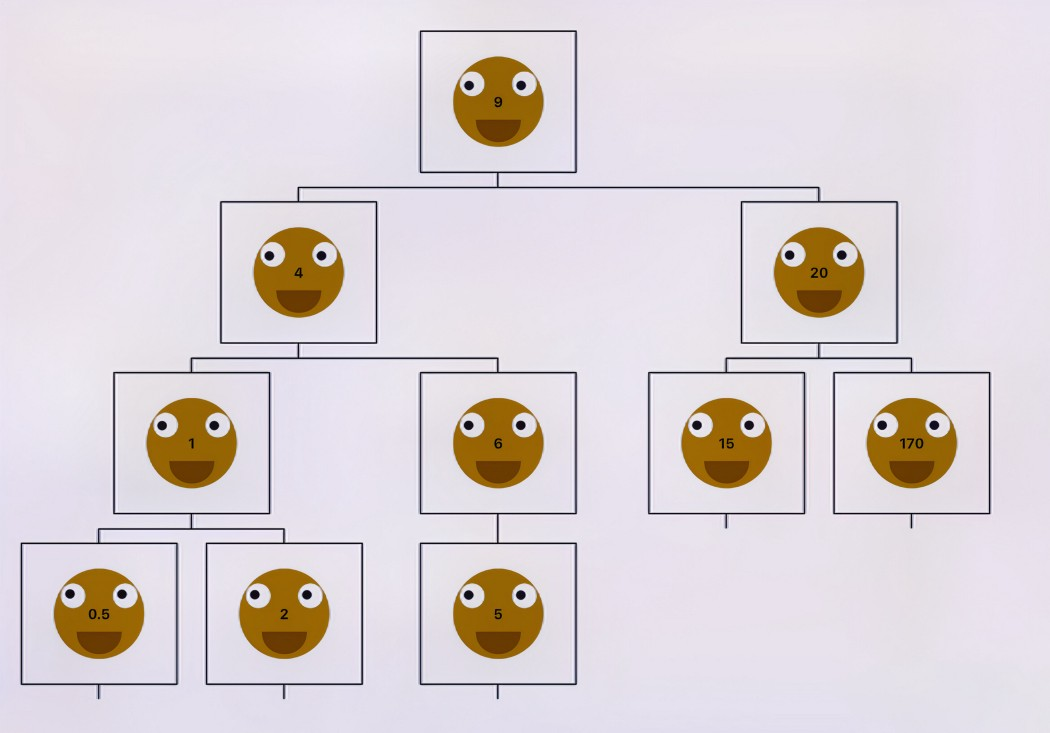#### Perfect Binary Tree

All nodes in a perfect binary tree have exactly two children and all the leaf nodes in the tree are on the same level. The first Binary tree Data Structure image posted above describes a perfect binary tree.

#### Binary Search Tree

A binary Search tree is a special type of tree data structure that can come in the form of any of the above binary trees. The binary search tree’s uniqueness is that the nodes on its left have lesser values than the nodes on its right. All three images shown above are examples of binary search trees. This uniqueness makes it one of the most powerful data structures in computer science; thus, the rest of this article will be centered around why the Binary Search Tree.

## Binary Tree Operations

### The Insert Operation

The insert operation is the process of adding new nodes to a binary tree. The algorithm looks for the best available branch in the tree to insert a new node while maintaining the fundamental characteristics of a binary search tree; i.e, the left of the tree containing nodes of lesser value to that of its right.

The time complexity of the insert operation O(h) where h is the height of the tree.

### Look Up Operation

The look Up operation as the name implies entails checking if a node exists in a tree.

Remember the binary tree’s fundamental property? Well, the algorithm of a look-up operation can skip all nodes to the left or the right of the tree: this depends on whether the value of the node is greater or less than the value we are looking for. The look-up operation in a binary tree is very efficient in that we won’t have to go through each item in the tree during a look-up operation. The best-case time complexity of a lookup operation is O(log N) where N is the number of nodes in the tree.

### Delete/Remove Operation

This is the process of deleting a node from a Binary Search Tree While also ensuring that the binary tree remains balanced.

When A Parent node is deleted from a Binary Search Tree, its child nodes are rearranged and attached to a new parent in such a way that the tree still retains all its unique properties. The best time complexity of a remove/ delete operation is O(log N) where N is the number of nodes in the tree.

This is the process of traversing or iterating through each of the nodes of a Binary Search tree.

It starts at the root of the tree and explores next-level nodes from left to right or right to left. This process continues until the root of the tree is reached and all nodes have been interacted with. The time complexity of a Breadth-first search operation is O(N) where N is the number of nodes in the tree.

This is the process of traversing a tree to its deepest node. I.e when a node is visited, it is traversed to its leaf node before traversing a sibling node. There are 3 common methods in which this algorithm can be achieved.

### Dept First Search In Order Traversal

We will recursively traverse the left subtree for every node (n) starting from the root node, then we process (n) itself after all its left node has been processed before moving to the right nodes then repeat the same process.

In this type of traversal, the leftmost leaf node will be processed first why the rightmost leaf node will be processed last. The time complexity of inorder traversal is O(N) where N is the number of nodes in the tree.

### Dept First Search Pre Order Traversal

For every node (n) starting from the root node, we will process (n), then recursively traverse the left subtree of (n), before moving to the right nodes and repeating the same process.

In this type of traversal, the root node will be processed first why the rightmost leaf node will be processed last. The time complexity of preorder traversal is O(N) where (N) is the number of nodes in the tree.

### Dept First Search Post Order Traversal

We will recursively traverse the left subtree for every node (n) starting from the root node. Then, we recursively process the right subtree of (n) before processing (n).

In this type of traversal, the leftmost leaf node will be processed first while the root node will be processed last. The time complexity of post-order traversal is O(N) where (N) is the number of nodes in the tree.

## Advantages of Binary Search Tree

1. We can keep the time complexity of insertion, deletion, and lookup operations to O(log N) which can be very efficient for large data volumes.
2. It is good for storing hierarchical data.
3. It can be used to store large data volumes.
4. It helps maintain a sorted data set.
5. It can be used to implement various search algorithms.

## Disadvantages of Binary Search Tree

1. The major disadvantage of binary search trees is that we have to maintain the balance of the tree for its accuracy.

#### Conclusion

Here we have looked at binary trees in data structures, explored their types, operations, and benefits. Binary Trees are used for both searching and storing data. Thus, they play a key role in the study of Data Science and its applications. We will be discussing the technical implementation of the Binary Search Trees and their operations in my next post.

Resources

• The playground to check out the binary tree operations can be found here